#### Real algebraic numbers

As stated previously, the sequence of projections ends with a univariate polynomial over. The sides of the cells will be defined based on the precise location of the roots of this polynomial. Furthermore, representing a sample point for a cell of dimensionin a complex in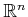for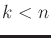requires perfect precision. If the coordinates are slightly off, the point will lie in a different cell. This raises the complicated issue of how these roots are represented and manipulated in a computer.

For univariate polynomials of degree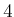or less, formulas exist to compute all of the roots in terms of functions of square roots and higher order roots. From Galois theory [469,769], it is known that such formulas and nice expressions for roots do not exist for most higher degree polynomials, which can certainly arise in the complicated semi-algebraic models that are derived in motion planning. The roots incould be any real number, and many real numbers require infinite representations.

One way of avoiding this mess is to assume that only polynomials in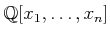are used, instead of the more general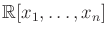. The field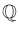is not algebraically closed because zeros of the polynomials lie outside of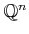. For example, if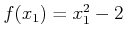, then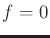for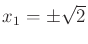, and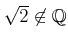. However, some elements ofcan never be roots of a polynomial in.

The set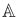of all real roots to all polynomials in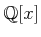is called the set of real algebraic numbers. The set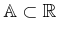actually represents a field (recall from Section 4.4.1). Several nice algorithmic properties of the numbers inare 1) they all have finite representations, 2) addition and multiplication operations on elements ofcan be computed in polynomial time, and 3) conversions between different representations of real algebraic numbers can be performed in polynomial time. This means that all operations can be computed efficiently without resorting to some kind of numerical approximation. In some applications, such approximations are fine; however, for algebraic decompositions, they destroy critical information by potentially confusing roots (e.g., how can we know for sure whether a polynomial has a double root or just two roots that are very close together?).

The details are not presented here, but there are several methods for representing real algebraic numbers and the corresponding algorithms for manipulating them efficiently. The running time of cylindrical algebraic decomposition ultimately depends on this representation. In practice, a numerical root-finding method that has a precision parameter,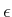, can be used by choosingsmall enough to ensure that roots will not be confused. A sufficiently small value can be determined by applying gap theorems, which give lower bounds on the amount of real root separation, expressed in terms of the polynomial coefficients . Some methods avoid requiring a precision parameter. One well-known example is the derivation of a Sturm sequence of polynomials based on the given polynomial. The polynomials in the Sturm sequence are then used to find isolating intervals for each of the roots . The polynomial, together with its isolating interval, can be considered as an exact root representation. Algebraic operations can even be performed using this representation in time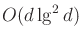, in which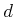is the degree of the polynomial . See [77,173,852] for detailed presentations on the exact representation and calculation with real algebraic numbers.

Steven M LaValle 2020-08-14